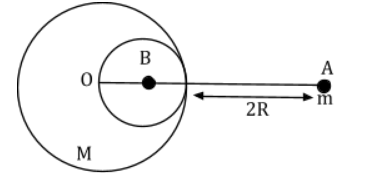# A solid sphere of radius R gravitationally attracts a particle placed at 3R from its centre with a force F1. Now a spherical cavity of radius R/2 is made in the sphere (as shown in figure) and the force becomes F2. The value of F1: F2 is:

A solid sphere of radius R gravitationally attracts a particle placed at 3R from its centre with a force F1. Now a spherical cavity of radius R/2 is made in the sphere (as shown in figure) and the force becomes F2. The value of F1: F2 is:a. 41 : 50

b. 36 : 25

c. 50 : 41

d. 25 : 36

Gravitational field intensity g1 = GM/(3R)2 = GM/9R2 …(1)

Gravitational field intensity g2 = GM/9R2 – G(M/8)/(5R/2)2

= GM/9R2 – GM/R250

= (41/9×50) GM/R2….(2)

Implies , g1/g2 = 41/50

⇒ F1/F2 = mg1/mg2 = 41/50(0)(6)